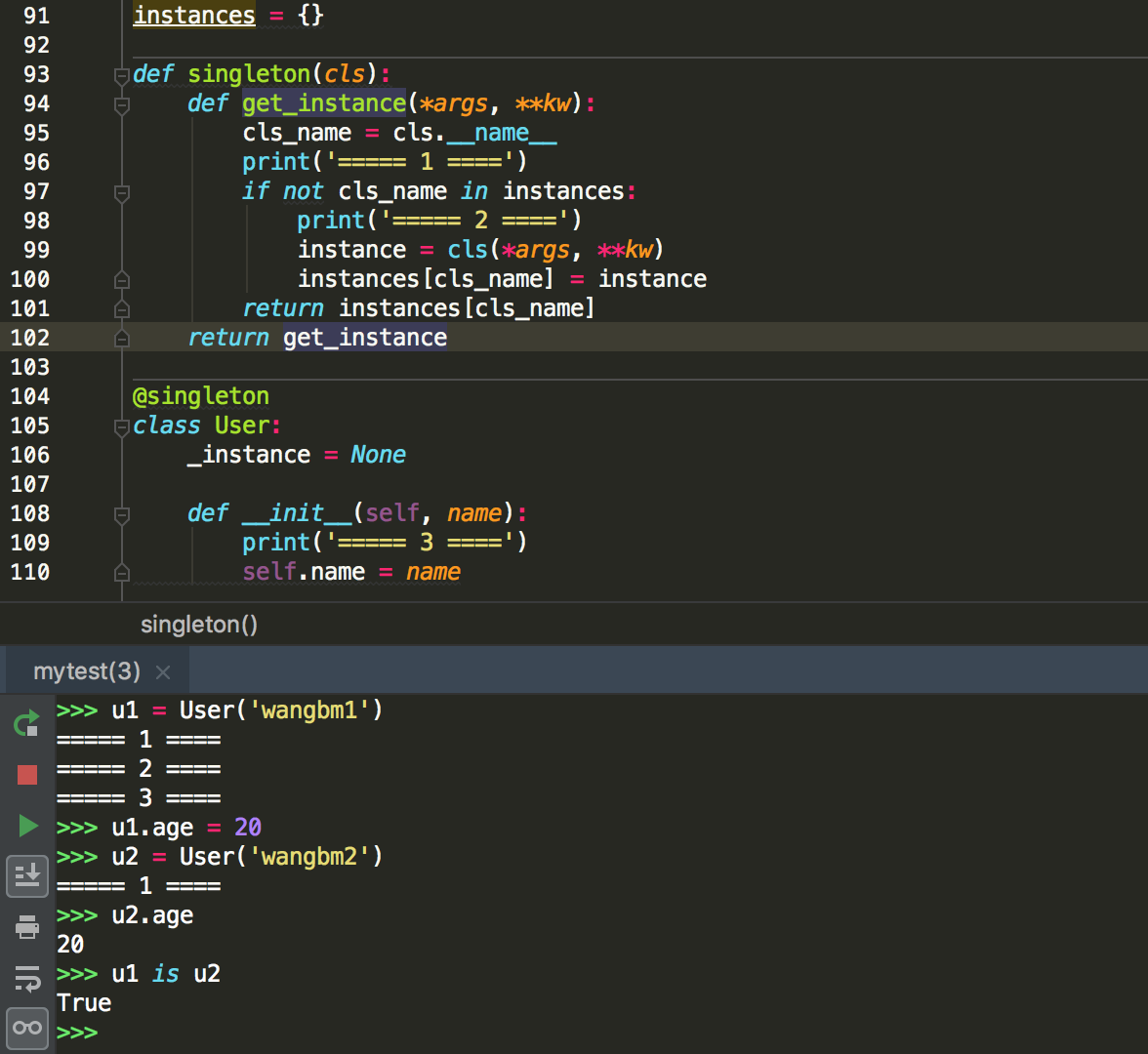# 3.10 Python装饰器的六种写法¶• 先定义一个装饰器（帽子）

• 再定义你的业务函数或者类（人）

• 最后把这装饰器（帽子）扣在这个函数（人）头上

```# 定义装饰器
def decorator(func):
def wrapper(*args, **kw):
return func()
return wrapper

# 定义业务函数并进行装饰
@decorator
def function():
print("hello, decorator")
```

• 更加优雅，代码结构更加清晰

• 将实现特定的功能代码封装成装饰器，提高代码复用率，增强代码可读性

## 1. 第一种：普通装饰器¶

• 在函数执行前，先记录一行日志

• 在函数执行完，再记录一行日志

```# 这是装饰器函数，参数 func 是被装饰的函数
def logger(func):
def wrapper(*args, **kw):
print('我准备开始执行：{} 函数了:'.format(func.__name__))

# 真正执行的是这行。
func(*args, **kw)

print('主人，我执行完啦。')
return wrapper
```

```@logger
def add(x, y):
print('{} + {} = {}'.format(x, y, x+y))
```

```add(200, 50)
```

```我准备开始执行：add 函数了:
200 + 50 = 250

```

## 2. 第二种：带参数的函数装饰器¶

```@periodic_task(spacing=60)
def send_mail():
pass

@periodic_task(spacing=86400)
def ntp()
pass
```

```# 小明，中国人
@say_hello("china")
def xiaoming():
pass

# jack，美国人
@say_hello("america")
def jack():
pass
```

```def say_hello(contry):
def wrapper(func):
def deco(*args, **kwargs):
if contry == "china":
print("你好!")
elif contry == "america":
print('hello.')
else:
return

# 真正执行函数的地方
func(*args, **kwargs)
return deco
return wrapper
```

```xiaoming()
print("------------")
jack()
```

```你好!
------------
hello.
```

## 3. 第三种：不带参数的类装饰器¶

```class logger(object):
def __init__(self, func):
self.func = func

def __call__(self, *args, **kwargs):
print("[INFO]: the function {func}() is running..."\
.format(func=self.func.__name__))
return self.func(*args, **kwargs)

@logger
def say(something):
print("say {}!".format(something))

say("hello")
```

```[INFO]: the function say() is running...
say hello!
```

## 4. 第四种：带参数的类装饰器¶

`__init__` ：不再接收被装饰函数，而是接收传入参数。 `__call__` ：接收被装饰函数，实现装饰逻辑。

```class logger(object):
def __init__(self, level='INFO'):
self.level = level

def __call__(self, func): # 接受函数
def wrapper(*args, **kwargs):
print("[{level}]: the function {func}() is running..."\
.format(level=self.level, func=func.__name__))
func(*args, **kwargs)
return wrapper  #返回函数

@logger(level='WARNING')
def say(something):
print("say {}!".format(something))

say("hello")
```

```[WARNING]: the function say() is running...
say hello!
```

## 5. 第五种：使用偏函数与类实现装饰器¶

```import time
import functools

class DelayFunc:
def __init__(self,  duration, func):
self.duration = duration
self.func = func

def __call__(self, *args, **kwargs):
print(f'Wait for {self.duration} seconds...')
time.sleep(self.duration)
return self.func(*args, **kwargs)

def eager_call(self, *args, **kwargs):
print('Call without delay')
return self.func(*args, **kwargs)

def delay(duration):
"""
装饰器：推迟某个函数的执行。
同时提供 .eager_call 方法立即执行
"""
# 此处为了避免定义额外函数，
# 直接使用 functools.partial 帮助构造 DelayFunc 实例
return functools.partial(DelayFunc, duration)
```

```@delay(duration=2)
def add(a, b):
return a+b
```

```>>> add    # 可见 add 变成了 Delay 的实例
<__main__.DelayFunc object at 0x107bd0be0>
>>>
>>> add(3,5)  # 直接调用实例，进入 __call__
Wait for 2 seconds...
8
>>>
>>> add.func # 实现实例方法
<function add at 0x107bef1e0>
```

## 6. 第六种：能装饰类的装饰器¶

```instances = {}

def singleton(cls):
def get_instance(*args, **kw):
cls_name = cls.__name__
print('===== 1 ====')
if not cls_name in instances:
print('===== 2 ====')
instance = cls(*args, **kw)
instances[cls_name] = instance
return instances[cls_name]
return get_instance

@singleton
class User:
_instance = None

def __init__(self, name):
print('===== 3 ====')
self.name = name
```